Vol. 45
Latest Volume
All Volumes
All Issues
0000-00-00
PIER
Vol. 45, 313-333, 2004
Near Field and Surface Field Analysis of Thin Wire Antenna in the Presence of Conducting Cube
X. Zhang A. Liu Yee Hui Lee Arokiaswami Alphones Chang-Hong Liang
The electric field integral equation (EFIE) with the method of moments (MoM) is used for treating problems of a thin wire antenna in the presence of a conducting cube. Pulse-expansion and point-matching technique in MoM are applied to both thin wire and closed object. For simplicity and efficiency, hybrid method for calculating near field and more importantly the surface field of objects is presented. Several examples in this paper show the validity of the proposed pulse-expansion and point-matching technique and the simplified hybrid calculation method.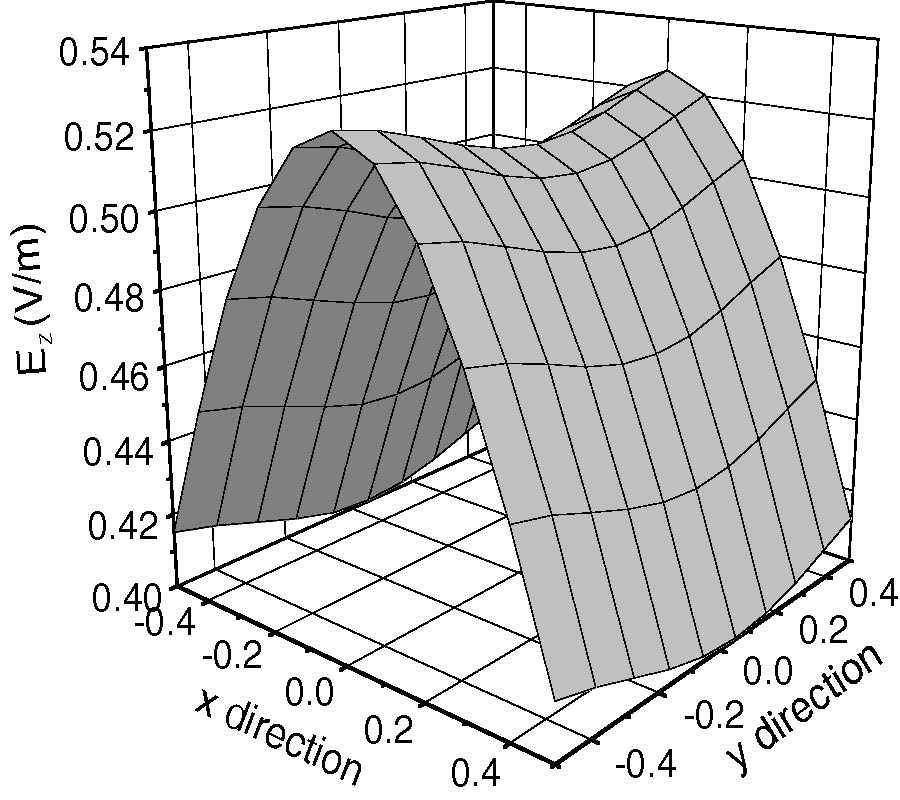0000-00-00
PIER
Vol. 45, 291-312, 2004
Solution of Time Domain Pmchw Formulation for Transient Electromagnetic Scattering from Arbitrarily Shaped 3-D Dielectric Objects
Baek-Ho Jung Tapan Kumar Sarkar Young-Seek Chung
In this paper, we analyze the transient electromagnetic response from three-dimensional (3-D) dielectric bodies using a time domain PMCHW (Poggio, Miller, Chang, Harrington, Wu) integral equation. The solution method in this paper is based on the Galerkin's method that involves separate spatial and temporal testing procedures. Triangular patch basis functions are used for spatial expansion and testing functions for arbitrarily shaped 3-D dielectric structures. The time domain unknown coefficients of the equivalent electric and magnetic currents are approximated by a set of orthonormal basis functions that are derived from the Laguerre functions. These basis functions are also used as the temporal testing. Use of the Laguerre polynomials as expansion functions characterizing the time variable enables one to handle the time derivative terms in the integral equation and decouples the space-time continuum in an analytic fashion. We also propose an alternative formulation using a differential form of time domain PMCHW equation with a different expansion for the equivalent currents. Numerical results computed by the two proposed methods are presented and compared.0000-00-00
PIER
Vol. 45, 277-289, 2004
The Variational Closed-Form Formulae for the Capacitance of One Type of Conformal Coaxial Lines
Chang-Hong Liang Xiao-Wei Shi Juan Yang
The variational closed-forms of the capacitance formulae of a type of conformal coaxial lines are presented in this paper with the assumption that the equipotential lines are conformal to the contour of the coaxial line. The variational extreme formula of the functional of continuous functions is first obtained. Then the variational stable and analytical expression of the capacitance is deduced. Considering the actual applications, we give the variational stable formulae of the capacitances of the conformal coaxial transmission lines whose contours are homogeneous curves of the 1st, 2nd and n-th order. Examples are given including the conformal regular polygonal, elliptical and highorder elliptical coaxial lines.0000-00-00
PIER
Vol. 45, 263-275, 2004
Wenquan Che Edward Yung Ke Wu Xiaodong Nie
In this paper,the design idea and technique of the ferrite phase shifter in substrate integrated waveguide (SIW) has been reported. The characteristics of the millimeter-wave ferrite phase shifter have been firstly calculated analytically,and the relative parameters of the device have been decided on the basis of optimization. At the same time,the design procedure of transition from ferrite phase shifter in SIW to CPW has been introduced; the agreement between the simulation results of the final integrated structure and the experimental results of an equivalent model has shown the good performance of this integrated structure.0000-00-00
PIER
Vol. 45, 243-262, 2004
Modal Method Analysis of Multilayered Coated Circular Waveguide Using a Modified Characteristic Equation
Fu-Gang Hu Chao-Fu Wang Yuan Xu Yeow-Beng Gan
In this paper, the modal method is applied to analyze coated circular waveguide terminated by a perfect electric conductor (PEC) plate. The key to this method is the accurate calculation of the propagation constants of modes in coated circular waveguide. To overcome numerical difficulties, such as overflow, encountered in solving characteristic equation, the characteristic equation is modified using Hankel function of the second kind instead of Bessel function of the first kind in the coated layers. The modified characteristic equation can be accurately solved to obtain the propagation constants even for very large circular waveguide with highly lossy coatings. To verify the modified characteristic equation, the attenuation and scattering property of circular waveguide structure have been simulated. Simulation results agree well with the reference results.0000-00-00
PIER
Vol. 45, 217-241, 2004
Penetration by a Laser-Light Induced Field and Coupling to a Monopole Attached on Axis to a Body of Revolution
Cengiz Ozzaim Chalmers Butler
In this paper is presenteda methodfor computing, andan experimental procedure for verifying, the coupling of a signal, caused by a modulatedlaser beam, to a loadimp edance terminating a coaxial waveguide whose center conductor protrudes into a an open-ended body of revolution (BOR). The excitation is the signal radiated by electrons emittedfrom the conducting surface by an impinging laser beam, modulated in such a way that the electrons escaping the surface oscillate harmonically in time causing them to radiate a coherent signal at an angular frequency Ï‰. For a vanishingly small spot of laser light on the conducting surface, the radiating source is modeled as an electric dipole normal to and located at the surface. To perform this computation directly is very difficult so we resort to an indirect methodthat allows us to realize significant savings with no loss in generality. The indirect approach adopted here takes the advantage of the reciprocity andallo ws one to determine the receivedsignal at the coax terminal load from knowledge of the field radiated by a small wire probe mounted on the symmetry axis of the BOR under the condition that the excitation results from a current generator impressedat the terminal endof the coax. This scheme necessitates the formulation andsolution of a simpler integral equation. In principle, the approach developed to solve this problem is exact and rigorous. The validity of this approach is demonstrated numerically and experimentally.0000-00-00
PIER
Vol. 45, 201-215, 2004
Wave Propagation and Reflection in the Ionosphere. an Alternative Approach for Ray Path Calculations
Stergios Isaakidis Thomas Xenos
In this work,the ray trajectory for oblique incidence is determined by solving the wave equation using the Finite Element Method (FEM). In case of ionospheric reflection the Discrete Fourier Transform (DFT), applied here in the space domain, is used to discriminate the incident and reflected waves. Ray tracing equations are combined with the Poynting vector components in order to calculate the wave trajectory taking into account the Earth's curvature. The results are illustrated for different frequencies and angles of incidence using electron density profiles obtained from the Rome digisonde station,topside profiles from the IRI-95 model and atmospheric data from the MSIS-E-90 model of NSSDC as an inputs.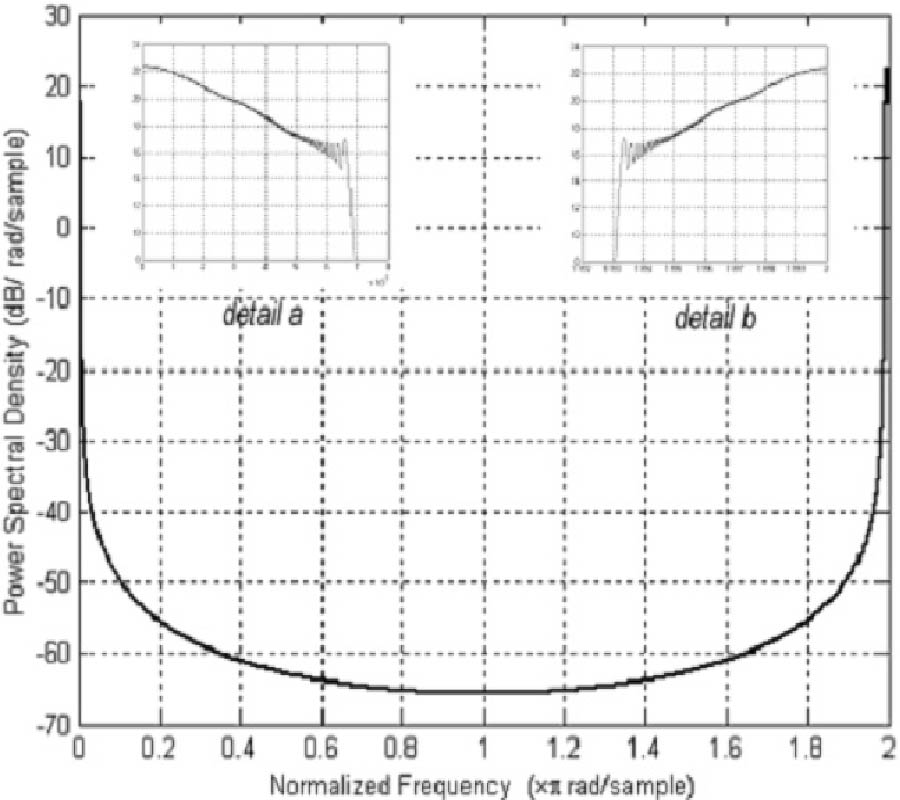0000-00-00
PIER
Vol. 45, 181-199, 2004
Mixed Potential Spatial Domain Green's Functions in Fast Computational Form for Cylindrically Stratified Media
Jin Sun Chao-Fu Wang Joshua Le-Wei Li Mook-Seng Leong
A new procedure for fast computing mixed potential spatial domain Green's functions for cylindrically stratified media is developed in this paper. Based on the fundamental behaviour of electric field Green's functions, spectral domain Green's functions for mixed potential integral equation (MPIE) are formulated by decomposing electric field Green's functions into appropriate forms. The spatial domain mixed potential Green's functions are obtained by using inverse Fourier transform applied to the spectral domain Green's functions. The summations of infinite cylindrical harmonics are accelerated by subtracting a term to resolve the problem of the series' slow convergence and by using the Shank's transform. The Sommerfeld integrals are efficiently evaluated using the discrete complex image method (DCIM) and the generalized pencil of function (GPOF) technique.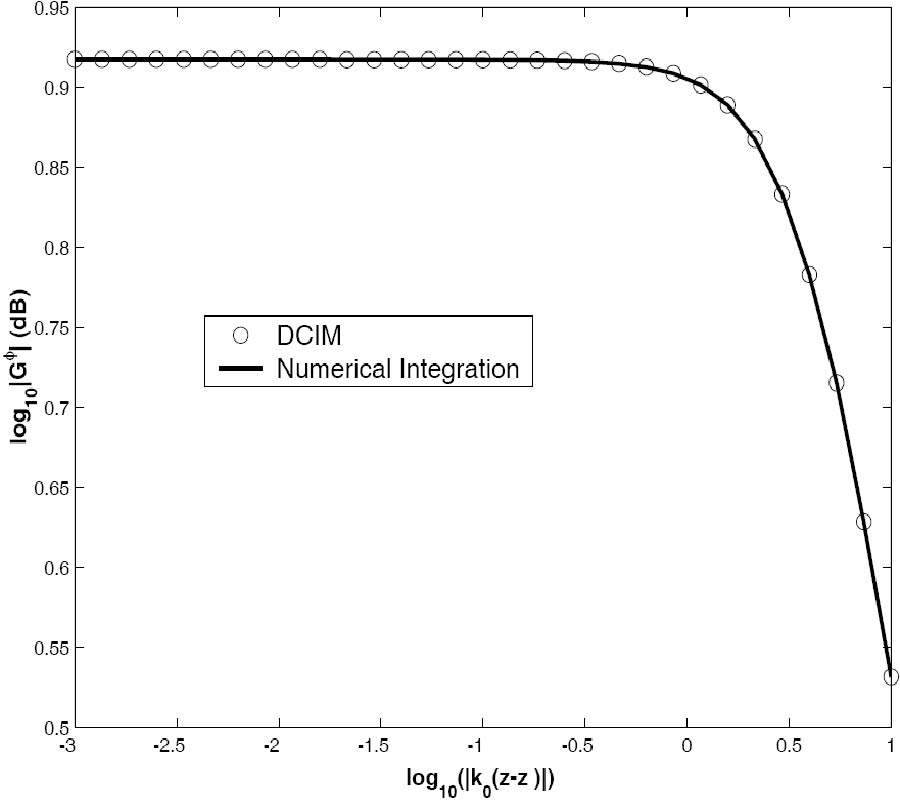0000-00-00
PIER
Vol. 45, 153-180, 2004
Non-Relativistic Boundary Conditions and Scattering in the Presence of Arbitrarily Moving Media and Objects: Cylindrical Problems
Dan Censor
Recently non-relativistic boundary conditions, based on the Lorentz force formulas, have been investigated. It was shown that to the first order in the relative velocity v/c the results for scattering problems are in agreement with the exact relativistic formalism. Examples for scattering by material objects moving in free space have been discussed. Presently the feasibility of non-relativistically solving scattering problems involving arbitrary material media is investigated. For concreteness, two representative canonical problems were chosen: scattering by a uniformly moving circular cylinder, and the related problem of a cylinder at rest, comprised of a uniformly moving medium in the cylindrical cross-sectional plane. The investigation demonstrates that solving such problems is feasible, and indicates the complexity involved in such an analysis. The main highlights are that we need to evaluate the phases and amplitudes of waves at the scatterer's surface, employing formulas based on the Lorentz force formulas and the Fresnel drag concept. The explicit solutions for the scattering problem display velocity-dependent interaction of the scattering coefficients.
0000-00-00
PIER
Vol. 45, 123-151, 2004
Performance Trends of on-Chip Spiral Inductors for Rfics
S. Pan Wen-Yan Yin Joshua Le-Wei Li
Abstract-Performance trends varying with different geometrical parameters for on-chip spiral inductors: (a) with fixed inner-dimension, (b) with outer-dimension, and (c) variation in both inner- and outerdimensions are extensively investigated in this paper. The relationships for the inductance, Q-factor and self-resonance frequency (SRF) with various geometrical parameters, such as track width, track spacing, and turn numbers are examined on an extensive experiment basis. These performance trends can be a good guideline for practical inductor designs in RFICs.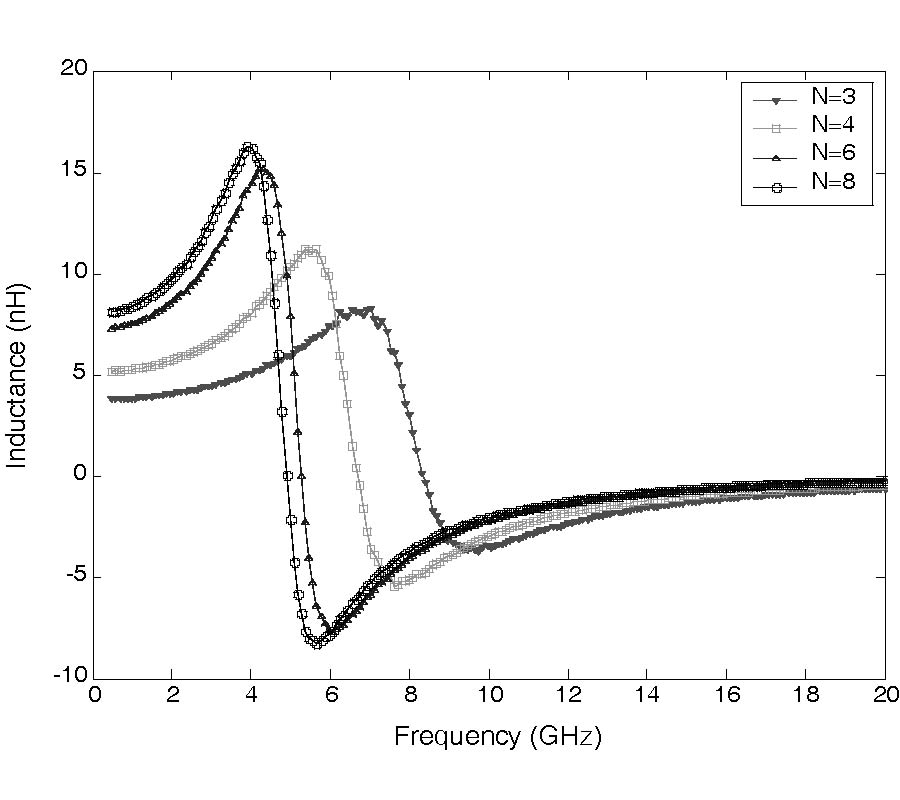0000-00-00
PIER
Vol. 45, 103-121, 2004
Electromagnetic Field in the Presence of a Three-Layered Spherical Region
Kai Li Seong-Ook Park Hong-Qi Zhang
Abstract-In this paper, the region of interest consists of the spherical dielectric earth, coated with a dielectric layer under the air. The simple explicit formulas have been derived for the electromagnetic fields of a vertical electric dipole and vertical magnetic dipole in the presence of three-layered spherical region, respectively. Next, basing on the above results, the formulas for the six components of the field in the air generated by a horizontal electric dipole are derived by using reciprocity. The computations show that the trapped surface wave of electric type can be excited efficiently and the trapped surface wave of magnetic type can not be excited when the thickness l of the dielectric layer is somewhat and satisfies the condition 0 < âˆš(k21-k20)*l < Ï€/2.When the thickness l of the dielectric layer is somewhat and satisfies the condition Ï€/2 < âˆš(k21-k20)*l < Ï€, the trapped surface wave of magnetic type can be excited. These formulas and the computations can be applied to the surface communications at the lower frequencies.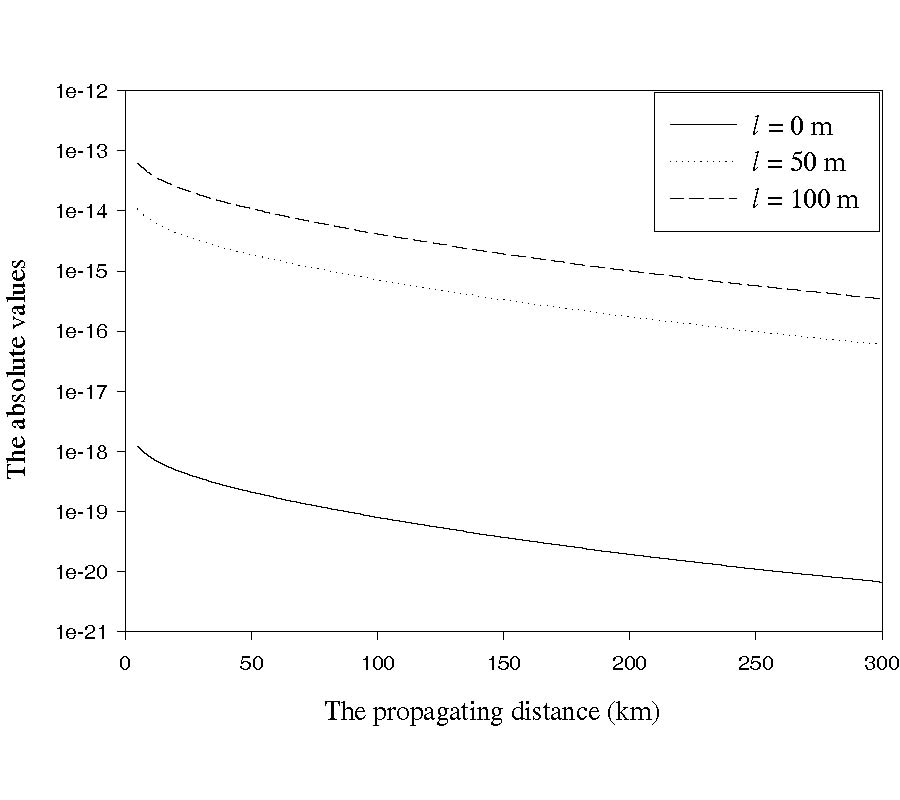0000-00-00
PIER
Vol. 45, 77-102, 2004
Diffraction by a Terminated Semi-Infinite Parallel Plate Waveguide with Two-Layer Material Loading and Impedance Boundaries
Metin Dumanli
The plane wave diffraction by a terminated semi infinite parallel-plate waveguide with two-layer material loading and impedance boundaries is rigorously analyzed for E polarization using the Wiener-Hopf technique. Introducing the Fourier transform for the scattered field and applying boundary conditions in the transform domain, the problem is formulated in terms of the simultaneous Wiener-Hopf equations, which are solved via the factorization and decomposition procedure. The scattered field is evaluated by taking the inverse Fourier transform and applying the saddle point method. The numerical examples of the radar cross section (RCS) are represented for various physical parameters and backscattering characteristics, of considered geometry for open ended cavity and discussed in detail.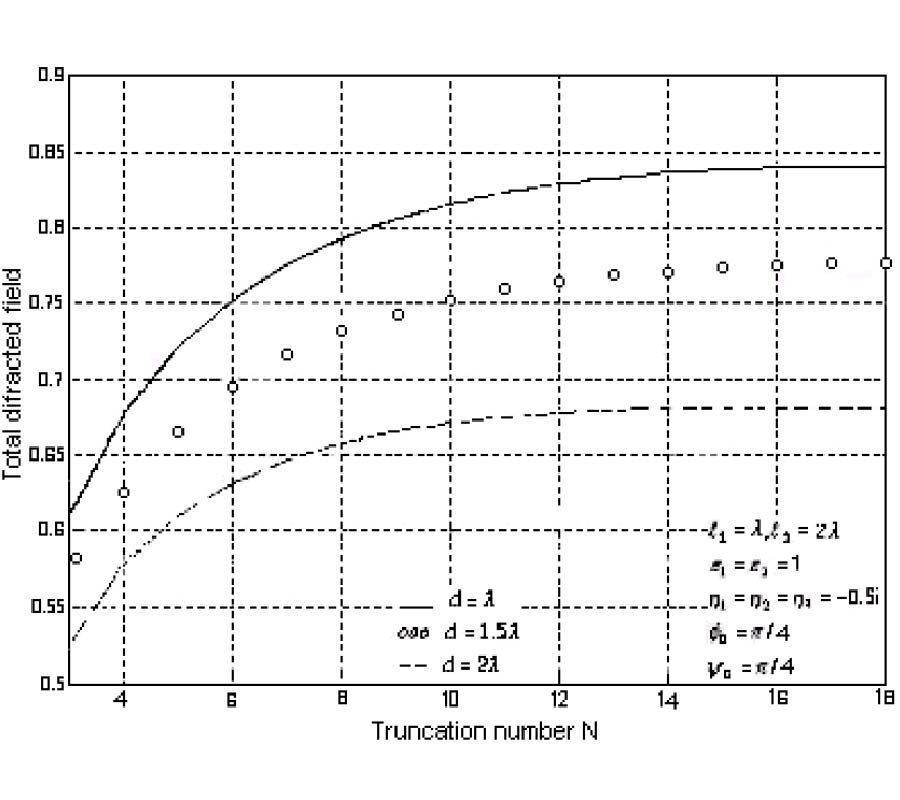0000-00-00
PIER
Vol. 45, 45-75, 2004
An Approximate Solution for Skew Incidence Diffraction by an Interior Right-Angled Anisotropic Impedance Wedge
Giuliano Manara Paolo Nepa Giuseppe Pelosi Andrea Vallecchi
The scattering by an anisotropic impedance interior rightangled wedge is analyzed when the principal anisotropy directions on the two faces are parallel and perpendicular to the edge. The problem is first approached by directly applying geometrical optics (GO); this allows us to identify the conditions under which the edge diffracted contribution vanishes. For those configurations not satisfying the above conditions, a perturbative technique, based on the Sommerfeld-Maliuzhinets method, is developed to determine an approximate edge diffracted field solution, valid when the normalized surface impedances on the anisotropic faces assume small values. The perturbative corrections to the field are asymptotically evaluated in the context of the Uniform Geometrical Theory of Diffraction (UTD).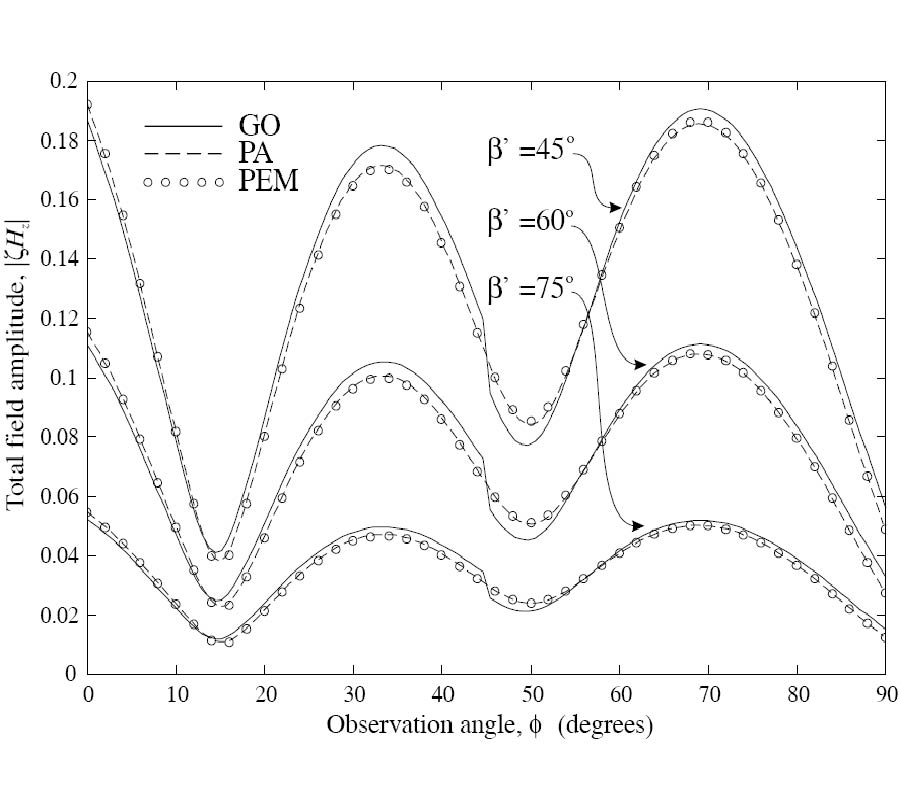0000-00-00
PIER
Vol. 45, 21-44, 2004